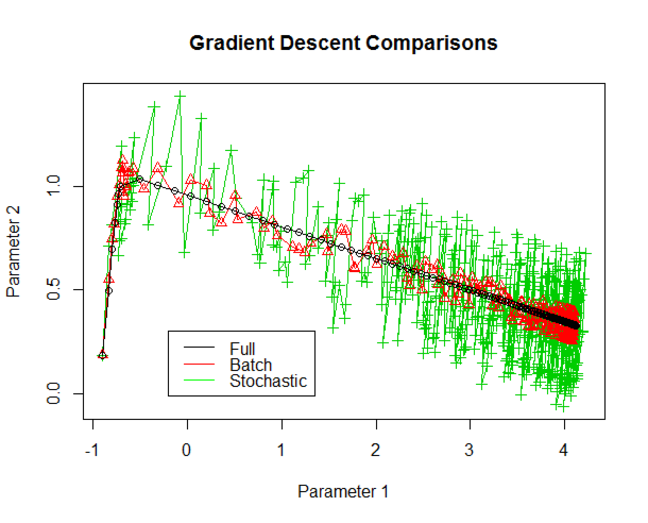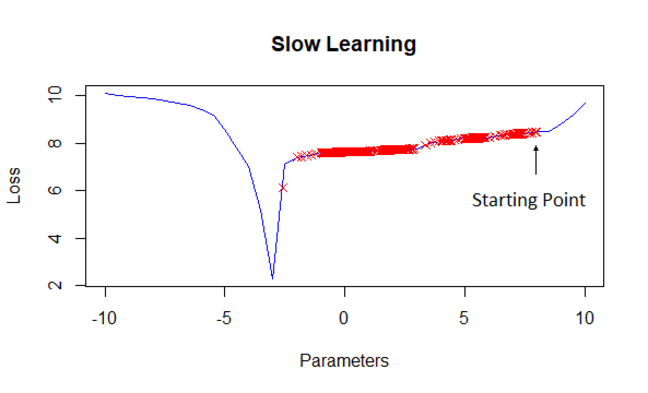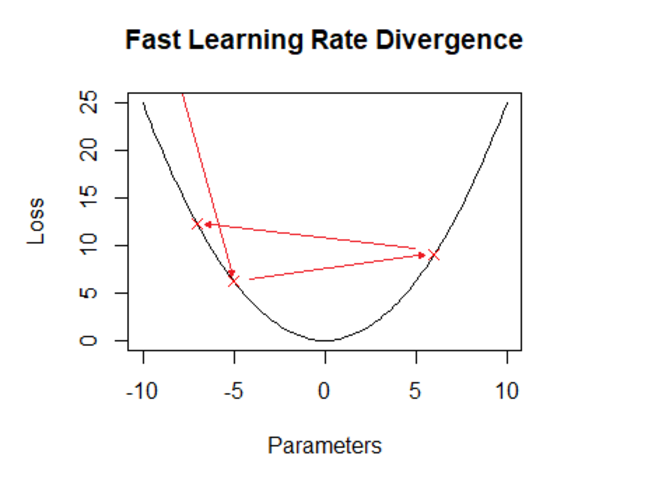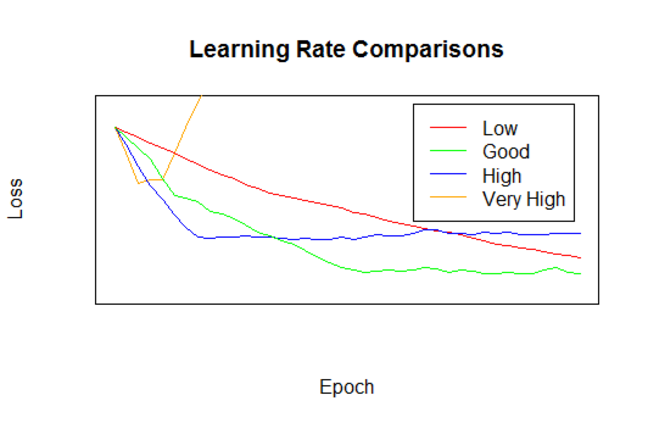## Want to keep learning?

This content is taken from the The Open University & Persontyle's online course, Advanced Machine Learning. Join the course to learn more.
2.21

# Training

We have seen that a basic neural network is a series of feature transformations culminating in either linear regression (for regression problems) or logistic regression (for classification problems). Both these transformations and the final linear/logistic regression output are parameterized by the weights in the network. The task of training the network is one of optimizing these weights given the data.

As we saw in the first module, this process involves the specification of a loss function which is then minimized. Depending on the loss function, this may be achieved in different ways. Regarding neural networks, the overwhelmingly most common approach is to utilize some form of gradient descent.

Common loss functions are mean square error for regression, and cross-entropy for classification:

$$L_{MSE}(\beta,X,Y)=\frac{1}{N}\sum_{i=1}^{N}(f(\beta,X_i)-Y_i)^2$$ $$L_{CE}(\beta,X,Y)= \sum_{i=1}^{N} -Y_i \cdot log(f(\beta,X_i)) - (1-Y_i)\cdot log(1-f(\beta,X_i))$$

In the cross-entropy loss, the Y is assumed to be binary labels 0 and 1. It is very popular in training deep neural networks for classification tasks.

#### Aside: Cross-entropy

Minimizing the cross entropy seeks to minimize the Kullback Liebler divergence (a measure of divergence between two probability distributions) between the empirical distribution (from the data) and the predicted distribution (from the model). Entropy, cross-entropy and the Kullback Liebler divergence and concepts from information theory.

Given a loss function, the basic gradient descent algorithm is the same as that given in week one. Before beginning, we specify a constant learning rate, R, or a learning rate schedule, R(i), where i is the step of the algorithm is specified. We assume a constant rate for simplicity.

1. Assign random initial values to the weights. These should be close to, but not precisely, zero. The weight cannot be uniformly assigned zero because the weight gradients will all be zero at this point and gradient descent will not go anywhere!

2. Repeat until convergence:

i. For each weight, $$w_*$$, calculate its partial derivative with respect to the Loss function, $$\frac{\partial L}{\partial w_*}$$.

ii. Update each weight given its partial derivative and the learning rate: $$w_* = w_* - \frac{\partial L}{\partial w_*} \cdot R$$

#### Aside: Backpropagation

You may have heard of backpropagation. This is a means of efficiently calculating the partial derivatives of the weights in the network. The name comes from the fact that the partial derivatives are calculated backwards through the network using the chain rule of derivation.

### Stochastic & Mini-Batch Gradient Descent

Running the simple algorithm is problematic. The loss functions as defined are functions of the whole data set. This means that every iteration of the algorithm (every update to the weights) requires calculating the estimated value for the target variable for every datum. Since there will be many iterations of the algorithm this could be a very long process.

Stochastic and mini-batch gradient descent are two methods of responding to this problem. Rather than calculating the loss function over the entire training data at each iteration, the loss function is calculated over some subset, known as a batch. This is mini-batch gradient descent. The extreme case when the loss function is calculated only for a single datum each iteration is known as stochastic gradient descent. Accordingly, when you train a neural network you are often asked to specify a batch size to be used in this way.

Chosing a batch size, however, involves a trade off. The smaller the batch size the quicker the gradient descent iterations are to run. But equally, the smaller the batch size the more noisy the minimization progress will be. While following the derivatives for the loss function calculated on the entire data set will be sure to point in a direction reducing the loss function on the data, the derivation calculated on an individual datum or small subset of the data may be inaccurate, as seen in the diagram below.### Epochs

Notice that the gradient descent algorithm looks at the data repeatedly. In the basic algorithm specified above, we continue until convergence is reached. If each step using one $$\frac{1}{n}$$ of the data, there is no guarantee that convergence will be reached in $$n$$ steps. So we will have to cycle through the data more than once. When training networks, it is common to be asked for the maximum number of times that you want to cycle through the data. This is known as the number of epochs. When proceeding from one epoch to another, the data is normally shuffled (reordered in a random order) leading to the use of different subsets as batches.

### Learning Rate

We note that before beginning the gradient descent algorithm we should choose a learning rate or learning schedule. Here we discuss what a learning schedule is and why it can be useful.

Consider that the loss function for many datasets will contain (i) large areas of almost flat gradient corresponding to poor values for the model parameters; and (ii) high quality local optima at the ‘bottom’ of steep inclines. We are likely to start our optimization in an area of the first sort, and wish to end it in an area of the second sort.

Working with a slow learning rate, it will take a long time to tranverse the almost flat, low quality areas. This is because the gradient of these areas is small, and the iterations are the product of the gradient and learning rate. Having a fast learning rate in this area would be useful in avoiding wasting time. We might also be able to jump over shallow, low quality local optima that might exist in such an area.

But working with a fast learning rate can cause difficulties in small areas of steep gradient. At worst, the gradient descent algorithm may jump right out of the small areas of interest. If that does not occur, it may well still keep jumping over the nadir of the indentation. This may lead to the learning algorithm taking a long time to converge or failing to converge at all.

Since we expect a fast learning rate to be advantageous in early iterations, and a slow one to be advantageous in later iterations, it makes sense to have a learning rate that slows down as the algorithm progresses. We can do this with a learning schedule.In addition to specifying a learning schedule, additional ways of adjusting the learning rate are sometimes used. An example is the use of momentum, which will adjust the size of the adjustment to the weights in a step of the learning algorithm based on the size of the previous step. There are various other approaches that seek to work with an adaptive learning rate which will adjust the learning rate for individal parameters. See here for a simple overview of some of these approaches.

### Initialization of Weights

There are a number of ways to randomly initialize the weights in a network, from simple approaches that use Gaussian distributions with 0 mean and small variance, to more complicated initialization schemes such as Xavier initialization, which seeks to keep the amount of variance equal in different layers. The interested student can read more about Xavier initialization here.

### Stopping Training

The basic specification of the gradient descent algorithm continues until convergence. In fact, doing so will often lead to overfitting, even when regularization is performed (see next step). Accordingly, it is normal to periodically test the performance of a network being trained on hold-out validation data. Most quality neural network implementations will allow you to save a copy of the network parameters periodically during the learning process. This means that you can examine the performance of the network on the validation data, and recover the values of the parameters when this performance peaked.

Choosing a maximum number of epochs will, of course, also provide a termination criterion for the algorithm. Given the above, you want to make sure you choose enough epochs that you are able to find the point at which performance peaks on the validation data.